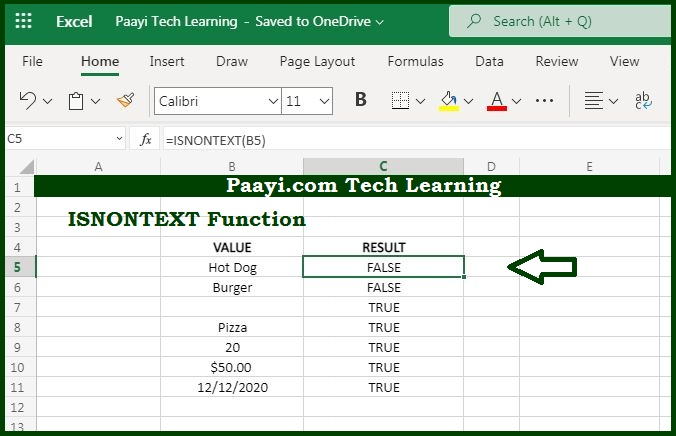# Learn How to Use Microsoft Excel ISNONTEXT Function

Written by | 0 Comments | 580 Views

In this article, you will learn how to use the Microsoft Excel IANONTEXT function and its prime function in Microsoft Excel. You will also get to know the Microsoft Excel ISNONTEXT function return value and syntax with the help of some examples.

Microsoft Excel ISNONTEXT Function

The main function of the Microsoft Excel ISNONTEXT function is to test for a non-text value. That means with the help of the ISNONTEXT function you can able to get the return the value as TRUE for non-text value like - numbers, dates, time, and so on. The ISNONTEXT function also returns TRUE for blank cells and cells containing formulas that return non-text results.

Return Value of ISNONTEXT Function

The return value will be logical TRUE or FALSE.

Syntax of ISNONTEXT Function

=ISNONTEXT(value)

Where the arguments:

• value: It is the value to check.

### How to Use Microsoft Excel ISNONTEXT Function?The main function of the Microsoft Excel ISNONTEXT function is to if the value is non-text. With the ISNONTEXT function, you can able to get the return value as the TRUE for non-text values like - numbers, dates, time, and so on. The ISNONTEXT function also returns TRUE for blank cells and cells containing formulas that return non-text results.

It should be noted that ISNONTEXT is part of a group of functions called the "IS functions", which returns logical values TRUE or FALSE.